IMO Shortlist 2014 problem C7

Kvaliteta:
Avg: 0,0
Težina:
Avg: 9,0

Let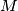$M$ be a set of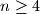$n \geq 4$ points in the plane, no three of which are collienar. Initially these points are connected with$n$ segments so that each point in$M$ is the endpoint of exactly two segments. Then, at each step, one may choose two segments$AB$ and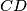$CD$ sharing a common interior point and replace them by the segments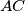$AC$ and$BD$ if none of them is present at this moment. Prove that it is impossible to perform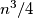$n^3 / 4$ or more such moves.

(Russia)

Izvor: https://www.imo-official.org/problems/IMO2014SL.pdf